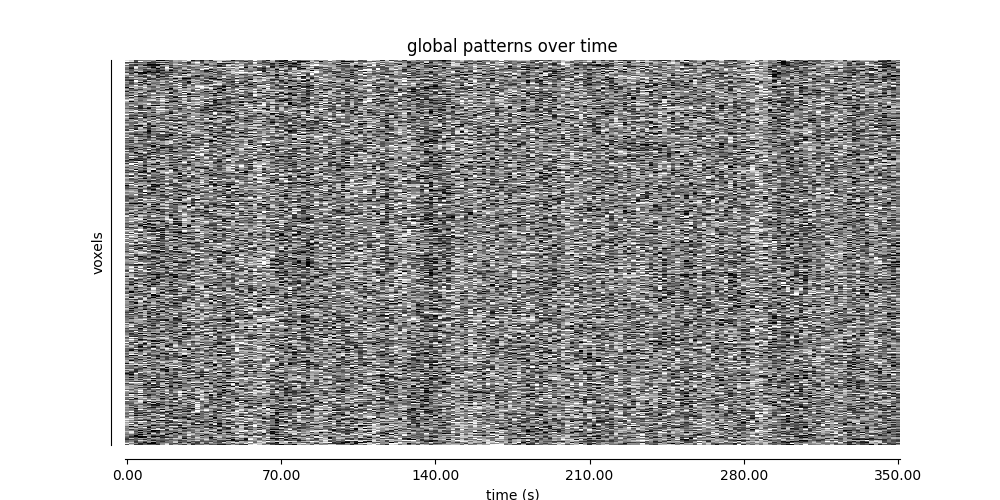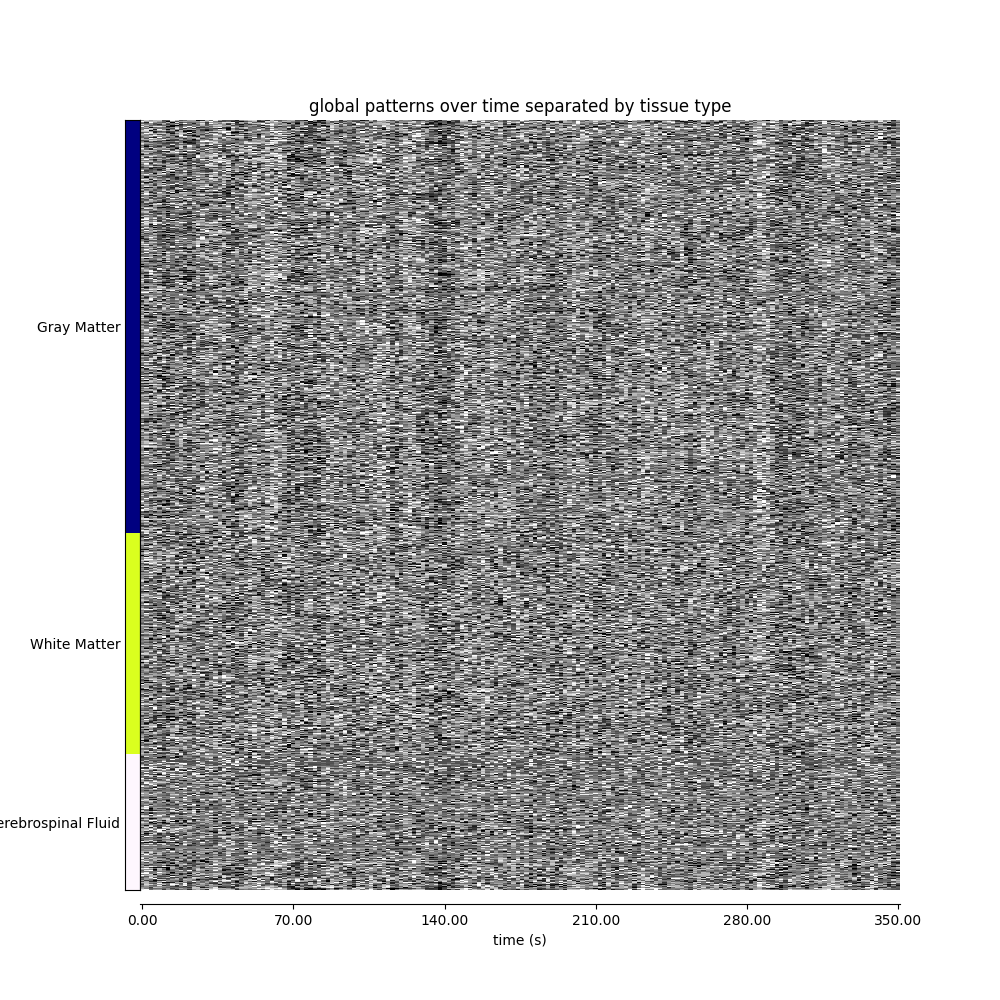# Visualizing global patterns with a carpet plot#

A common quality control step for functional MRI data is to visualize the data over time in a carpet plot (also known as a Power plot or a grayplot).

The `nilearn.plotting.plot_carpet` function generates a carpet plot from a 4D functional image.

## Fetching data from ADHD dataset#

```from nilearn import datasets

# plot_carpet can infer TR from the image header, but preprocessing can often
# overwrite that particular header field, so we will be explicit.
t_r = 2.0

# Print basic information on the dataset
print(
f"First subject functional nifti image (4D) is at: {adhd_dataset.func}"
)
```
```First subject functional nifti image (4D) is at: /home/runner/work/nilearn/nilearn/nilearn_data/adhd/data/0010042/0010042_rest_tshift_RPI_voreg_mni.nii.gz
```

```from nilearn import masking

# Build an EPI-based mask because we have no anatomical data
```

## Visualizing global patterns over time#

```import matplotlib.pyplot as plt

from nilearn.plotting import plot_carpet

display = plot_carpet(
t_r=t_r,
standardize="zscore_sample",
)

display.show()
```Create a gray matter/white matter/cerebrospinal fluid mask from ICBM152 tissue probability maps.

```import numpy as np

from nilearn import image

atlas = datasets.fetch_icbm152_2009()
atlas_img = image.concat_imgs((atlas["gm"], atlas["wm"], atlas["csf"]))
map_labels = {"Gray Matter": 1, "White Matter": 2, "Cerebrospinal Fluid": 3}

atlas_data = atlas_img.get_fdata()
discrete_version = np.argmax(atlas_data, axis=3) + 1
discrete_version[np.max(atlas_data, axis=3) == 0] = 0
discrete_atlas_img = image.new_img_like(atlas_img, discrete_version)
```
```/home/runner/work/nilearn/nilearn/examples/01_plotting/plot_carpet.py:68: UserWarning: Data array used to create a new image contains 64-bit ints. This is likely due to creating the array with numpy and passing `int` as the `dtype`. Many tools such as FSL and SPM cannot deal with int64 in Nifti images, so for compatibility the data has been converted to int32.
discrete_atlas_img = image.new_img_like(atlas_img, discrete_version)
```

## Visualizing global patterns, separated by tissue type#

```from nilearn.plotting import plot_carpet

fig, ax = plt.subplots(figsize=(10, 10))

display = plot_carpet(
discrete_atlas_img,
t_r=t_r,
axes=ax,
cmap="gray",
standardize="zscore_sample",
)

fig.show()
``````/usr/share/miniconda3/envs/testenv/lib/python3.9/site-packages/nilearn/image/resampling.py:591: UserWarning: Casting data from int32 to float32
warnings.warn(f"Casting data from {data.dtype.name} to {aux}")
Coercing atlas_values to <class 'int'>
```

Total running time of the script: (0 minutes 8.372 seconds)

Estimated memory usage: 1024 MB

Gallery generated by Sphinx-Gallery